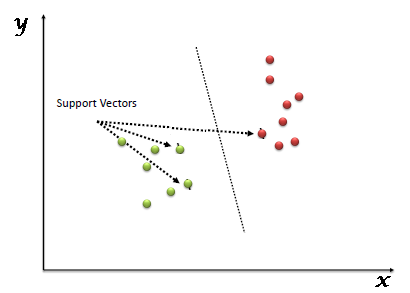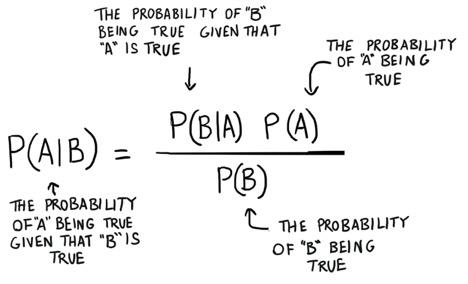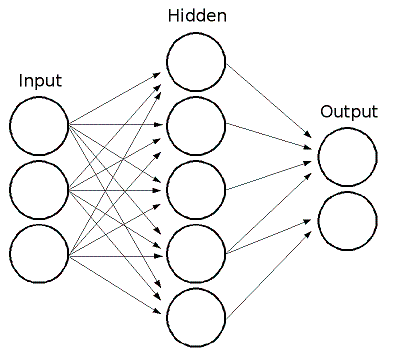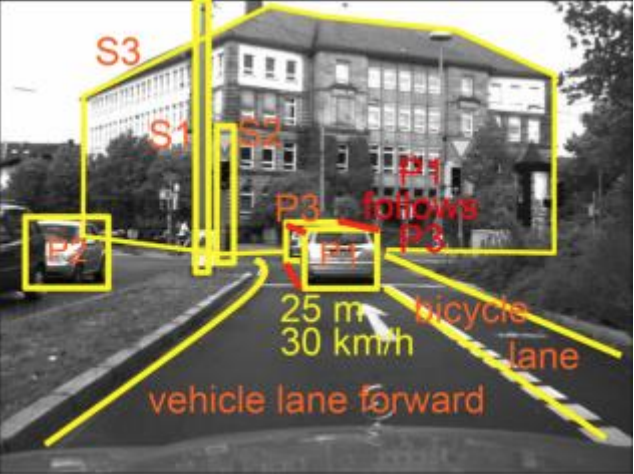6 Machine Learning Algorithms to Kickstart your Data Science EffortsWe cover a lot of topics here at GAP – whether it be big data and analytics, data science or even how to optimize your software delivery projects using agile or nearshore business models. In this blog post, we revisit data science. Specifically, we take a look at some of the most popular machine learning algorithms, often used when starting out in the area. After you’ve read this, you’ll be well versed in some of the most commonly used machine learning algorithms available out there.

Want the short version? Download our free “6 Machine Learning Algorithms” cheat sheet!

Before we jump into the algorithms, it’s worth pointing out that most machine learning algorithms can be classified as Supervised or Unsupervised learning, and recognizing which category fits your efforts is essential:

Supervised Learning

Supervised learning is where you have input variables (x) and an output variable (Y) and you use an algorithm to learn the mapping function from the input to the output. These algorithms are ideal for classification and regression tasks..

Y = f(X)

The goal is to approximate the mapping function so well that when you have new input data (x), you can predict the output variables (Y) for that data.

It is called supervised learning because the process of an algorithm learning from the training dataset can be thought of as a teacher supervising the learning process. We know the correct answers, the algorithm iteratively makes predictions on the training data and is corrected by the teacher. Learning stops when the algorithm achieves an acceptable level of performance.

Unsupervised Learning

Unsupervised learning is where you only have input data (X) and no corresponding output variables.

The goal of unsupervised learning is to model the underlying structure or distribution in the data in order to learn more about the data. Clustering and feature selection tasks usually fall into this category.

This is called unsupervised learning because unlike supervised learning, there is no correct answers and there is no teacher. Algorithms are left to their own devices to discover and present the interesting structure in the data.

Source: Machine Learning Mastery

Decision Trees

Decision Trees and Random Forests are one of the most popular classification algorithms due to their simplicity.  a decision tree is a model that uses a set of rules to calculate a specific value, starting at a “root” decision and branching at each step towards a more specialized result.  For example, imagine you’d like to predict the likelihood that a person is male or female; in the screenshot below, you can see a simple example of how this could be modeled in a decision tree:Rules often follow an IF THEN ELSE pattern, for example, rules for the decision tree in the above screenshot may be represented as follows:

• If Height > 180 cm Then Male
• If Height <= 180 cm AND Weight > 80 kg Then Male
• If Height <= 180 cm AND Weight <= 80 kg Then Female

As far as algorithms go, decision trees can be ideal for classification use cases in applications (which involves categorical variables), although they can also handle regression tasks.

One of the key benefits of using decision trees to arrive at predictions is they are very easy to interpret and explain to non-technical staff, potentially providing additional insight on the data. They also require relatively little effort from users in terms of data preparation.

That said, decision trees can become unstable even with the smallest changes to the input datasets,  calculations can become complex very quickly, especially if outcomes are linked and there’s certain conditions that are hard to model in terms of a simple “IF THEN ELSE” pattern.

Random Forests are an ensemble learning method, in which multiple trees are created during the training process, and then their mean (regression) or mode (classification) is used as the final classification output.

Support Vector Machine

The support vector machine algorithm (or SVM) is up next.  This is a supervised learning algorithm which is often used in classification or regression challenges.  For the algorithm to do this, each data item is placed as a point in n-dimensional space (where n is the number of features you have) with the value of each feature being a given coordinate.

Next, classification is performed by finding the hyperplanes inside that space that differentiate the target attribute’s classes.  You can see a simple 2-class example of this in the screenshot below:The algorithm can be deployed in many use cases, for example, medical researchers presented evidence generated using SVM to help classify persons with and without common diseases and to help even detect pre-diabetes signals.  You can find out more about how the algorithm was deployed in this research paper here.

Want the short version? Download our free “6 Machine Learning Algorithms” cheat sheet!

KNN

Our third algorithm is KNN. K-nearest neighbors is a supervised algorithm which focuses on classification and regression problems. It’s one of the more simpler algorithms out there and works on the premise that:

similar things exist in close proximity

Or in plain English, similar things are near each other. This can also be referred to as distance, proximity or closeness, or to get more technical – calculating the distance between points on a graph.

In this algorithm, the data set is separated into different “clusters”, with all members in the cluster being similar to each other. The KNN algorithm is ideal for building “recommendation engines,” such as those found in online services such as Amazon, Netflix or YouTube. One example is rendering “customers like you bought X” or “you might like this movie/clip”.

Another example of KNN is used when predicting the creditworthiness of finance applications, identifying that consumers who are most likely to default will share similar characteristics.

Some benefits of the KNN algorithm are that it’s easy to explain and understand, it’s versatile and it has relatively high accuracy. This can mean, however, that it can be computationally expensive to run the algorithm, as it needs to store nearly all the training data used to arrive at predictions. It can also be sensitive to irrelevant fields in your data. High dimensionality in the data can be counteracted by using feature selection algorithms.

Another disadvantage worth pointing out is that KNN requires a target amount of clusters to be passed as a parameter, which might not always be known. In circumstances like this one, the DBSCAN algorithm can be a better alternative, as it uses threshold parameters such as the minimum nodes to be included in a new cluster for it to count as one.

Naïve Bayes Classification

Bayesian Classification is an algorithm that attempts to calculate the probability that an event will occur given that another event has already occurred.

The rule is written like this: p(A|B) = p(B|A) p(A) / p(B) and you can read more about the inner workings of each part of the equation here.The algorithm can be deployed in many use cases and yields reasonably accurate predictive results, as much as 80% in some cases. For example, products like Social Opinion deploy Bayesian classification to help classify social media data into several categories such as positive, negative and neutral (sentiment analysis.)

One of the main benefits of Bayesian classification is that if you can feed the algorithm good data, you’ll find the predictions it can arrive at will improve. Alternatively, if the prior information (or training data) is of low quality, your predictions can move in the wrong direction – garbage-in-garbage-out.

Linear and Multiple Regression

The Linear Regression algorithm shows you the relationship between two variables (the independent variable and dependent variable) and how the change in one variable impacts the other – specifically what happens to the dependent variable when the independent variable is changed.

Independent variable(s) are referred to as explanatory variables, as they explain what factors impact the dependent variable. The dependent variable is often referred to as the factor of interest.

It’s one of the fastest machine learning algorithms and requires minimal fine tuning and can be deployed to many use cases such as sales forecasting or risk assessment. For example, if a company has been tracking sales each month, linear regression can help predict what sales may be in the future months. For behaviors that don’t behave linearly, implementations of this sort of model usually can be easily tweaked to add degrees of freedom.

That said, one disadvantage of this algorithm is that due to ie being an algebraic model, it can only be used with numerical data, meaning categorical attributes have to be encoded prior to being ingested by the model.

Neural Networks

Our brain is a supercomputer, it can multitask, organize and process numerous types of information using a vast network of neurons.  The Neural Network mimics this neuron structure.

The neural network consists of layers of computational units, called neurons. Each layer can be connected to the other and is responsible for processing data until data can be classified and an outcome determined.Each neuron in the network multiplies an initial value by some weight then sums the results with other values being passed into the neuron, adjusting the output by the neurons’ bias and normalizing the output with an activation function.Neural networks have been used in self-driving car projects such as NVIDIA’S. This autonomous car initiative can process multiple inputs since there are multiple things to consider, such as pedestrians, other cars and buildings.

They can be trained in other use cases too. For example, a neural network can be trained to predict outputs that are expected for a given set of outputs. If you have a network that fits well in modeling a given sequence of values, it can then be used to predict future results. This makes the neural network a suitable choice when it comes to arriving at stock market predictions.

Want the short version? Download our free “6 Machine Learning Algorithms” cheat sheet!

Unlike most of the algorithms discussed above, neural networks are well-known examples of “black boxes”, this is because it can be difficult to trace how the neural network arrived at particular decisions.

Summary

In this article, we looked at six machine learning algorithms that can help you deploy predictive solutions into your business. Many of these share attributes and qualities with other, more specialized algorithms, making it easy to iterate on your efforts without requiring specific knowledge.

We’ve explored how they run at a high level and looked at some of their advantages and disadvantages. We hope that by reading this blog post, you’ve got more insight into how some machine learning algorithms work and how you might be able to use them.

Here at Growth Acceleration Partners, we have extensive expertise in many verticals. Our nearshore business model can keep costs down while maintaining the same level of quality and professionalism you’d experience from a domestic team.

Our Centers for Engineering Excellence in Latin America focus on combining business acumen with development expertise to help your business. We can provide your organization with resources in the following areas:

• Software development for cloud and mobile applications
• Data analytics and data science
• Information systems
• Machine learning and artificial intelligence
• Predictive modeling
• QA and QA Automation

If you’d like to find out more, then visit our website here.  Or if you’d prefer, why not arrange a call with us?